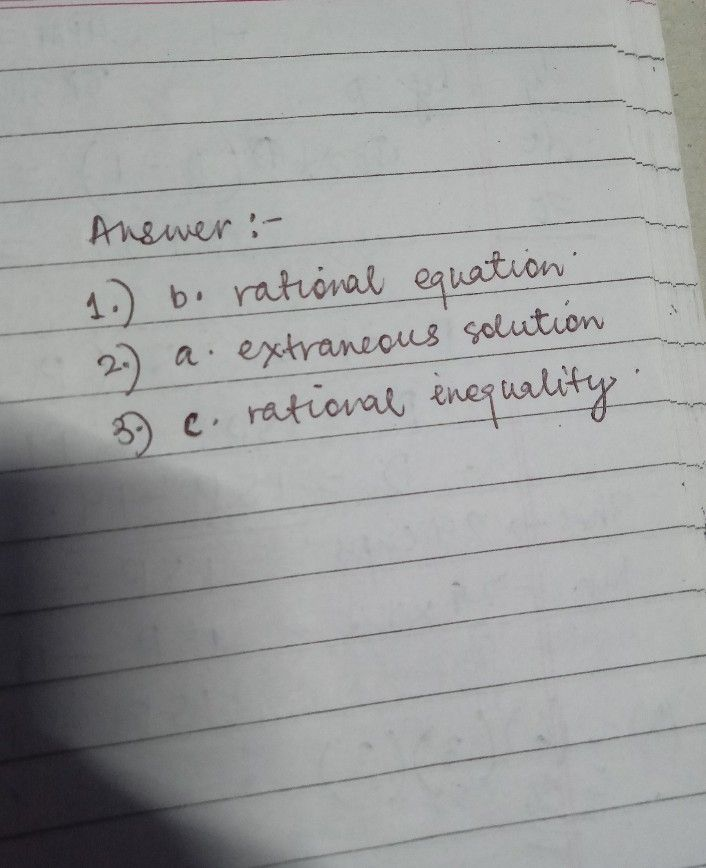Symbol
ProblemWhat $T$ Know $Choos$ the letter of the best answer. Write the chosen letter on a separate sheet of $P$ paper. $1.$ Which of the following expressions is an equality between two expressions containing one or more variables? a. rational function b. rational equation c. rational inequality d. irrational expression $2$ What do you call a root obtained in the process of solving an equation which looks correct but after analyzing it turns out as incorrect? a. extraneous solution b. rational expression $c.$ least common denominator $d$ quotient $3$ What do you call an inequality which involves one or more rational expressions? a. rational function b. rational equation $c.$ rational inequality $d.$ irrational expression $1$